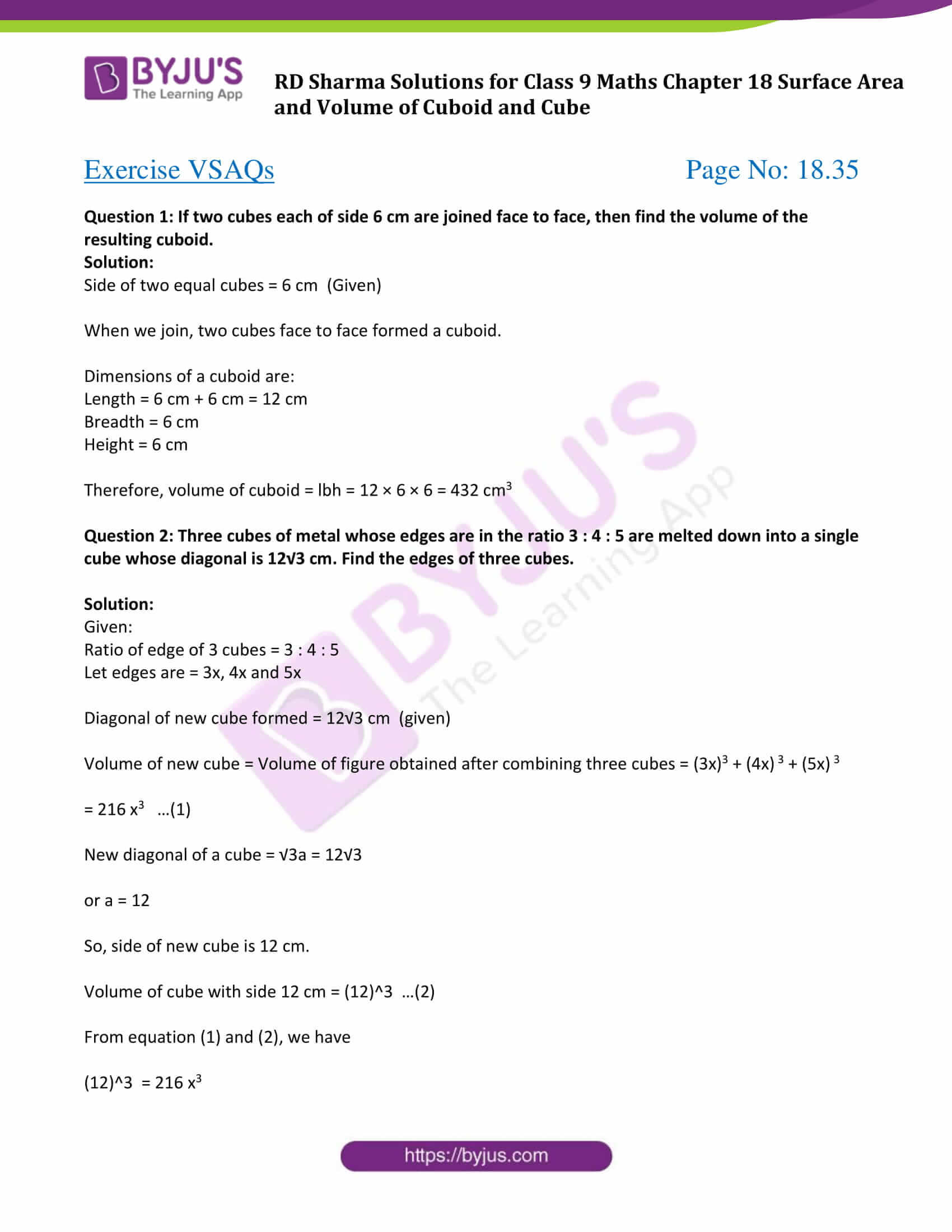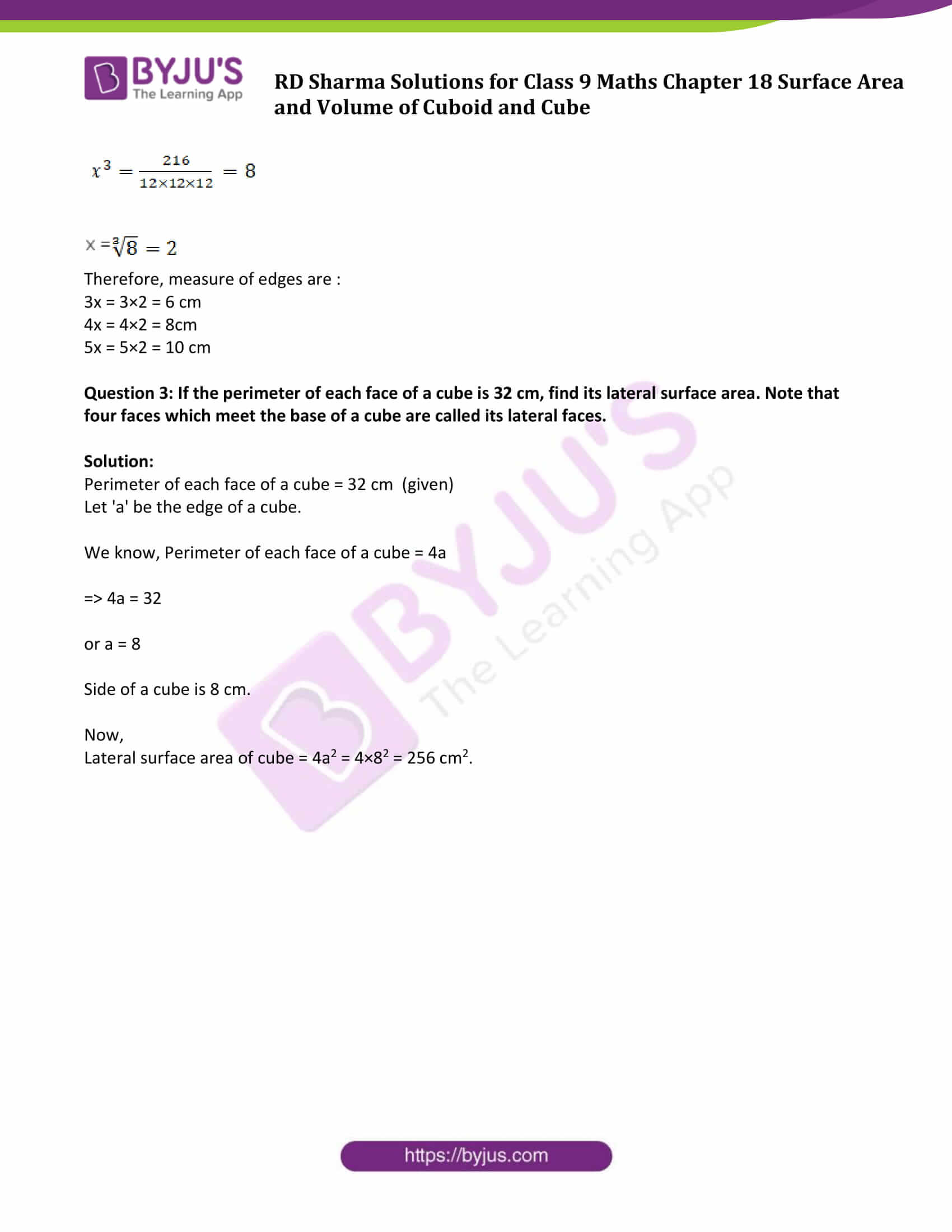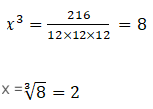# RD Sharma Solutions for Class 9 Maths Chapter 18 Surface Area and Volume of Cuboid and Cube Exercise VSAQs

Class 9 chapter 18 – Surface Area and Volume of Cuboid and Cube Exercise VSAQs solutions are provided only here. RD Sharma solutions for class 9 Maths includes all the questions provided in the textbook that is prescribed for 9th standard in accordance with the CBSE Board. This exercise questions help students to revise all concepts studied in this chapter. Students must have a strong conceptual knowledge in this subject which will be beneficial for them in their future studies. Hence, preparation of exams using the RD Sharma solutions will definitely provide students with an advantage of scoring well.

## Download PDF of RD Sharma Solutions for Class 9 Maths Chapter 18 Surface Area and Volume of Cuboid and Cube Exercise VSAQs### Access Answers to Maths RD Sharma Solutions for Class 9 Chapter 18 Surface Area and Volume of Cuboid and Cube Exercise VSAQs Page number 18.35

Question 1: If two cubes each of side 6 cm are joined face to face, then find the volume of the resulting cuboid.

Solution:

Side of two equal cubes = 6 cm (Given)

When we join, two cubes face to face formed a cuboid.

Dimensions of a cuboid are:

Length = 6 cm + 6 cm = 12 cm

Height = 6 cm

Therefore, volume of cuboid = lbh = 12 × 6 × 6 = 432 cm3

Question 2: Three cubes of metal whose edges are in the ratio 3 : 4 : 5 are melted down into a single cube whose diagonal is 12√3 cm. Find the edges of three cubes.

Solution:

Given:

Ratio of edge of 3 cubes = 3 : 4 : 5

Let edges are = 3x, 4x and 5x

Diagonal of new cube formed = 12√3 cm (given)

Volume of new cube = Volume of figure obtained after combining three cubes = (3x)3 + (4x) 3 + (5x) 3

= 216 x3 …(1)

New diagonal of a cube = √3a = 12√3

or a = 12

So, side of new cube is 12 cm.

Volume of cube with side 12 cm = (12)^3 …(2)

From equation (1) and (2), we have

(12)^3 = 216 x3Therefore, measure of edges are :

3x = 3×2 = 6 cm

4x = 4×2 = 8cm

5x = 5×2 = 10 cm

Question 3: If the perimeter of each face of a cube is 32 cm, find its lateral surface area. Note that four faces which meet the base of a cube are called its lateral faces.

Solution:

Perimeter of each face of a cube = 32 cm (given)

Let ‘a’ be the edge of a cube.

We know, Perimeter of each face of a cube = 4a

⇒ 4a = 32

or a = 8

Side of a cube is 8 cm.

Now,

Lateral surface area of cube = 4a2 = 4×82 = 256 cm2.

## RD Sharma Solutions for Class 9 Maths Chapter 18 Surface Area and Volume of Cuboid and Cube Exercise VSAQs

Class 9 Maths Chapter 18 Surface Area and Volume of Cuboid and Cube Exercise VSAQs is based on finding the surface area and volume of a cuboid and cube geometric figures. Students can download RD sharma Class 9 pdf to learn about three dimensional figures. Students will also learn how to find the surface area and volumes of these figures.